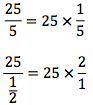Math is filled with theorems, axioms, and properties. Though the following properties may seem simplistic, the ISEE will couple these properties with difficult concepts, such as algebraic functions.

### Reflexive Property

A value is equal to itself.

• x = x

Examples

• 17 = 17
• y = 400

### Symmetric Property

Equal values may be placed on either side of the equals sign.

• x = y
• y = x

Examples

• x = 25 or 25 = x
• 5 = y or y = 5

### Transitive Property

If one value equals a second value and the second value equals a third value, then the third value equals the first.

• x = y
• y = z
• x = z

### Commutative Property

When solely adding values, you may change the order without changing the result. The same is true for multiplication.

• x + y = y + x
• x × y = y × x

Examples

• 1 + 2 + 3 = 6
• 3 +1 + 2 = 6
• 3 × 4 × 5 = 60
• 4 × 5 × 3 = 60

### Associate Property

When solely adding or multiplying values, you may change the groupings of values without changing the result. The groups are separated by parentheses.

•  x + (z) = (y) + z
• x × (× z) = (× y) × z

Examples

• 4 + (6 + 5) = (4 + 6) + 5
• 4 × (6 × 5) = (4 × 6) × 5

You must add the same value to both sides of the equals sign to maintain the equality of the values.

• x + a = y + a

Examples

• x + 2 = y + 2
• 5 + 3 = 5 + 3

### Multiplication Property

You must multiply the same value to both sides of the equals sign to maintain the equality of the values.

• ax = ay

Examples

• 4(20) = 4(20)
• 4(3-1) = 4(2)

### Distributive Property

You may multiply a value to a sum by first multiplying the value to the sum’s addends and then adding those products together.

• x(z) = xy + xz

Examples

• 5(3 + 7) = 5(3) + 5(7) = 15 + 35 = 50

### Definition of Division

When dividing values, it is the same thing as multiplying the value in the numerator by the reciprocal of the value in the denominator.

• x/y = x × 1/y
• x/(1/y) = x × y/1

Examples### Multiplication Property of Zero

The product of any value and zero will be zero.

• x × 0 = 0

Examples

• 15 × 0 = 0
• 12 × 11 × 10 × 0 = 0

### Zero Product Property

If the product of two values is zero, one of the values is zero.

• xy = 0
• x = 0 or y = 0

Examples

• 17x = 0; x = 0

### Definition of Zero as a Dividend

The quotient of zero and any value (except zero) is zero.

• 0/x = 0

Examples

• 0/9 = 0

### Definition of Zero as a Divisor

The quotient of any value and zero is considered undefined.

• x/0 = undefined

Examples

• 9/0 = undefined